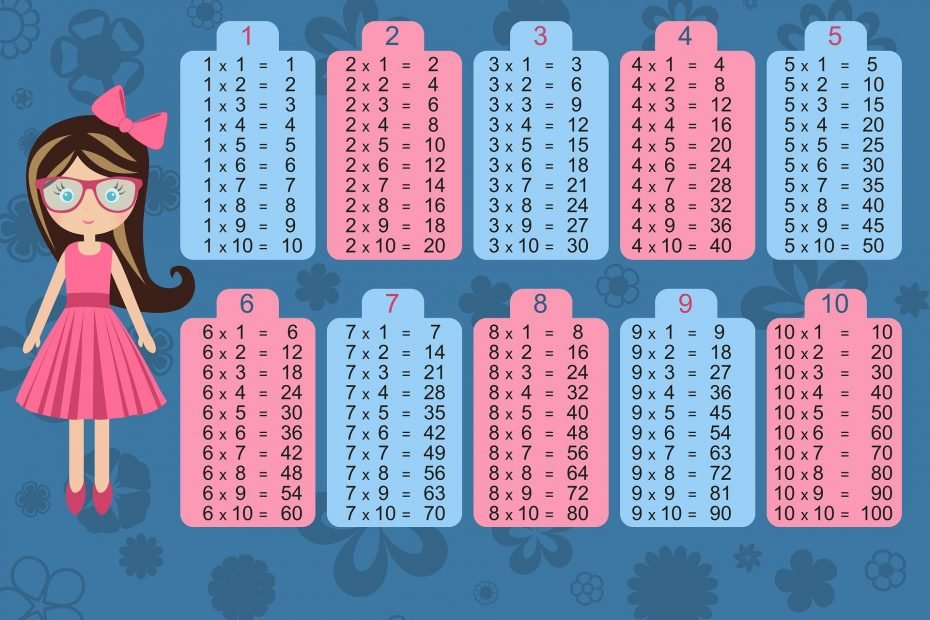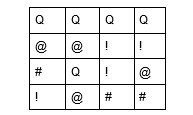Home » Blog » Maths Puzzles

# Maths PuzzlesLogic Puzzles

Check out this fun maths logic puzzle:Row 1 =24

Row 2 =28

Row 3 =23

Row 4 = 20

Column 3=19

Kids that have learned simultaneous equations should have no problem solving this puzzle. But many people cannot take a puzzle like this and know that simply using some basic equations can have you solve it super easily. Nevertheless, kids who have not yet learned simultaneous equations but love logic can solve the puzzle. Here’s how to solve it:

The first row tells us that 4Q=24, thus we know straight away Q=6

The second row tells us that 2@ + 2!=28 or 2(@+!)=28 therefore @+!=14

Since row 3 is @+!+Q+#=23 even though we don’t know the individual values of @+! We can still substitute in 14 and we can substitute in 6 for Q this means that 20+#=23 and #=3

Column 3 will now allow us to work out the value of ! simply substitute in #=3 and Q=6 so that you have 9+2!=19  therefore 2!=10 and !=5

Now substitute !=5 into @+5=14 so @=9

Check your values in other rows to know you have solved the puzzle successfully. Row 3 = 3+5+6+9=23

So:

Q=6

@=9

!=5

#=3

Correct! Well puzzled. Did you enjoy this puzzler? If your kids are not enjoying basic simultaneous equations, then creating puzzles like this with more boxes can be a great way for them to create equations rather than just solving them.

There is a set of rules for equations that are pretty basic. The LHS(left-hand side) must always equal the RHS. See, the use of brackets to simplify equations. We can change equations as long as we always do the same to the right and left-hand side, like we did in this cool puzzler.